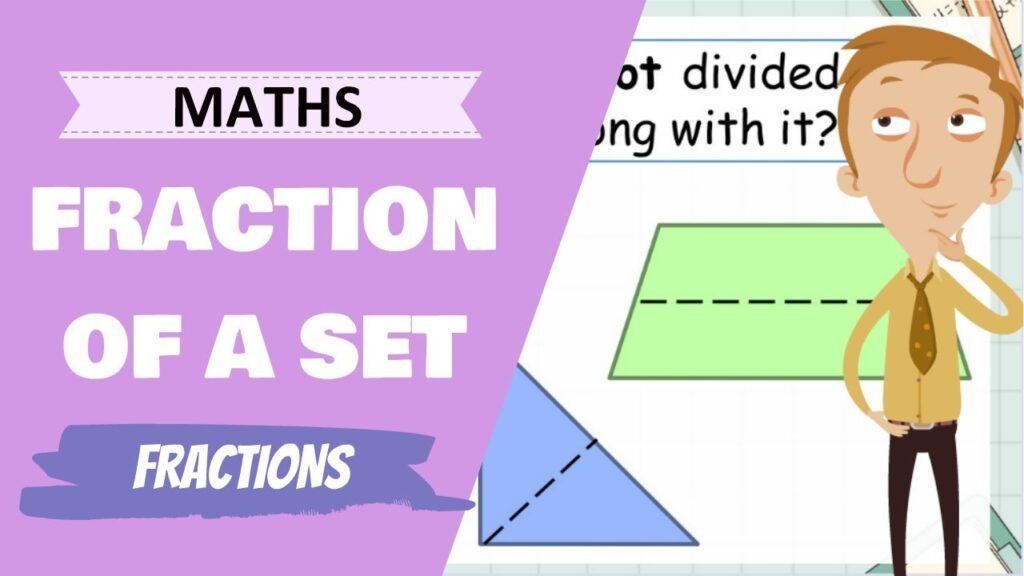# Fractions# Lessons and Activities

### Lesson 1

What are fractions?
In this lesson, we'll take a look at what we are actually measuring when we say fractions

### Lesson 2

Finding Fractions of Numbers
In this lesson, we'll take a look at how to find the fraction of a given number

### Lesson 3

Working with tenths
In this lesson, we'll take a look at what tenths look like and how to represent them

### Lesson 4

Finding the fraction of a set
In this lesson, we'll take a look at how to find the fraction of a set of objects

### Lesson 5

Fraction Walls
In this lesson, we'll take a look at what a fraction wall is and how we use them

### Lesson 6

Comparing Fractions
In this lesson, we'll take a look at how to compare and order fractions with different denominators

### Lesson 7

Fraction Word Problems
In this lesson, we'll take a look at how to solve word problems that involve fractions

### Lesson 8

Here's the Fraction... What is the number?
In this lesson, we'll take a look at how to find the whole number when given a fraction of it